﻿ Note on a Partition Function Which Assumes All Integral Values

### Note on a Partition Function Which Assumes All Integral Values

Manvendra TambaOPEN ACCESSPEER-REVIEWED

## Note on a Partition Function Which Assumes All Integral Values

Manvendra TambaDepartment of Mathematics, Goa University, Taleigao Plateau, Goa, India

### Abstract

Let G(n) denote the number of partitions of n into distinct parts which are of the form 2m, 3m, 5m, 6m-3, 8m-3, 9m-3 or 11m-3 with parts of the form 2m, 3m, 6m-3, or 11m-3 being even in number minus the number of them with parts of the form 2m, 3m, 6m-3, or 11m-3 being odd in number. In this paper, we prove that G(n) assumes all integral values and does so infinitely often.

• Tamba, Manvendra. "Note on a Partition Function Which Assumes All Integral Values." Turkish Journal of Analysis and Number Theory 2.6 (2014): 220-222.
• Tamba, M. (2014). Note on a Partition Function Which Assumes All Integral Values. Turkish Journal of Analysis and Number Theory, 2(6), 220-222.
• Tamba, Manvendra. "Note on a Partition Function Which Assumes All Integral Values." Turkish Journal of Analysis and Number Theory 2, no. 6 (2014): 220-222.

 Import into BibTeX Import into EndNote Import into RefMan Import into RefWorks

### 1. Introduction

Let S(n) denote the number of partitions of n into distinct parts with even rank minus the number with odd rank (see ). Andrew, Dyson and Hickerson  used the arithmetic of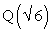to show that S(n) takes on every integral value infinitely often. This is the first time the interaction between the theory of partitions and algebraic number theory was exhibited. It was remarked in  that they know of no other partition function in the literature which assumes all integral values as S(n) does.

Let H(n) denote the number of partitions of n into parts which are repeated exactly 1, 3, 4, 6, 7, 9, or 10 times with the parts repeated exactly 1,4,6, or 9 times even in number minus the number of them with parts repeated exactly 1, 4, 6, or 9 times odd in number. In , using the arithmetic of Gaussian integers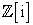, it was shown that H(n) assumes all integral values and does so infinitely often.

Let G(n) denote the number of partitions of n into distinct parts which are of the form 2m, 3m, 5m, 6m-3, 8m-3, 9m-3, or 11m-3 with parts of the form 2m, 3m, 6m-3, or 11m-3 being even in number minus the number of them with parts of the form 2m, 3m, 6m-3, or 11m-3 being odd in number. For example, G(7) is zero because (2(2)) + (3(1)), (2(2))+(6(1)-3) have even number of parts of the form 2m, 3m, 6m-3, or 11m-3. while (2(1))+(5(1)) and (2(1))+ (8(1)-3) areodd number of parts of the form 2m, 3m, 6m-3, or 11m-3 (here m-values are shown in bold). In this paper, we show that G(n) assumes all integral values and does so infinitely often.

### 2. Main Results

A For (positive) integer n, consider the equation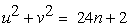(2.1)

We call a solution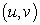of (2.1) admissible if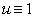(mod 6) and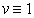(mod 6). For a (positive) integer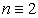(mod 24), let J(n) be the excess of the number of admissible solutions of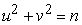with(mod 12) over the number of them with v not congruent to 1 modulo 12.

In subsequent sections, we shall be proving the following:

Therorem 1. For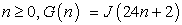.

Theorem 2. G(n) takes on every integer value infinitely often.

### 3. Proof of Theorem 1

First we note that the generating function of G(n) is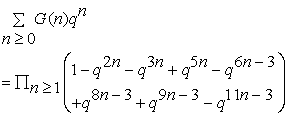Lemma 1. For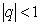,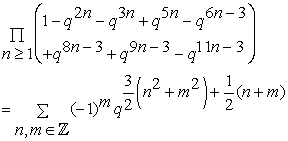Proof. Using Jacobi’s triple product identities (see , p. 21) we get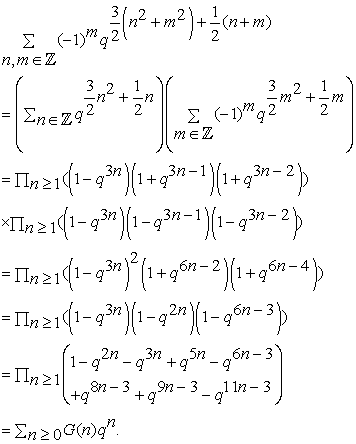This proves the Lemma.

Using this lemma, it follows that: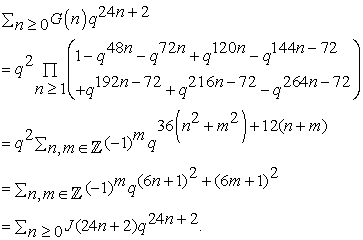This proves Theorem 1.

### 4. Arithmetic of J(n)

In this section we study J(n) using Gaussian integers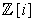, where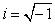. For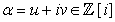, let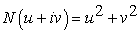. We define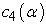in terms of u (mod 4) and v (mod 4) by

#### Table 1. Values of c4(α)

Let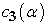be defined in terms of u ( mod 3) and v (mod 3) by the following table, where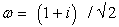:

#### Table 2. Values of c3(α)

Let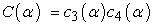and let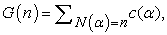where the sum is over a complex set non-associate Gaussian integers with norm n.

Let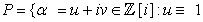(mod 6) and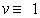(mod 6)} and let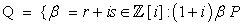or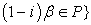. Then, for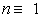(mod 12),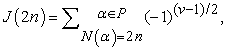(where v is the imaginary part of α)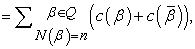(where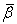is the conjugate of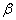)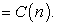Note that this together with Theorem 1 proves the assertion made in the Remark 2 of .

Thus we have shown that:

Lemma 2. For(mod 12),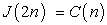.

Next we recall the properties of C(n) from .

Lemma 3. (a) The function C(n) is multiplicative.

(b)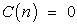unlessor 5 (mod 12).

Lemma 4. Let p be a prime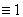(mod 12) and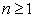. Then:

a. C(n) is either 0, 2 or -2.

b. If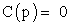, then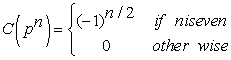c. If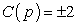, then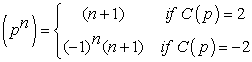Lemma 5. Let p be a prime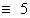(mod 12) and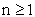be even. Then: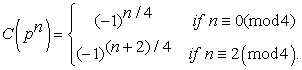### 5. Proof of Theorem 2

As in ,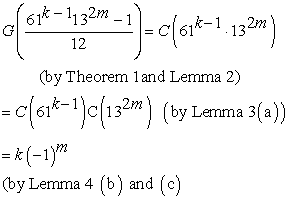and [, Table 1])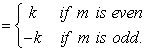This proves Theorem 2.

### 6. Conclusion

An arithmetical function f(n) is called lacunary if it is almost always 0 (see). In  it is shown that S(n) is lacunary. In  it is shown that H(n) is lacunary. So is is natural to ask whether G(n) is so. We make the following conjecture:

Conjecture. G(n) is lacunary.

### References

  G.E. Andrews, The Theory of Partitions, (G.-C. Rota, Ed.), Encyclopedia of Math. And its Applications, Vol. 2, Addison-Wesley, Reading, MA, 1976.In article  G.E. Andrews, Questions and conjectures in partition theory, Amer. Math. Monthly, 93 (1986) 708-711.In article CrossRef  G.E. Andrews, F.J. Dyson and D. Hickerson, Partitions and indefinitequadratic forms, Invent. math., 91 (1988) 391-407.In article  J. Lovejoy, Lacunary Partition Functions, Math. Research Letters, 9 (2002) 191-198.In article  M.Tamba, On a partition function which assumes all integral values, J.Number Theory 41 (1992) 77-86.In article CrossRef